# Impedance of Linear, Time-Invariant, Lumped-Element Circuits

The following relations are well worth committing to memory, as I will use them many times over during the course of this text. If they are not already familiar to you, see  , or any other general electrical engineering reference.

The impedance magnitude of a linear, time-invariant lumped-element inductor , as measured with a sinusoidal input at frequency f , is

Equation 1.1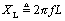where X L is the impedance magnitude (ohms), f is the measurement frequency (Hz), L is the inductance (Henries).

The impedance magnitude of a linear, time-invariant, lumped-element capacitor , as measured with a sinusoidal input at frequency f , is

Equation 1.2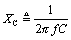where X C is the impedance magnitude (ohms), f is the measurement frequency (Hz), C is the capacitance (Farads).

When dealing with step waveforms (like digital signals), an appropriate frequency of analysis for estimating the effective impedance of a parasitic element, over a time scale corresponding to a rising (or falling) edge, is

Equation 1.3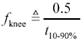where f knee is the assumed frequency for sinusoidal analysis (Hz), t 10 “90% is the 10% to 90% rise (or fall) time of the circuit (seconds).

The knee frequency , f knee , is a crude estimate of the highest frequency content within a particular digital signal (Figure 1.1). Signaling channels whose parasitic impedances are not significant at all frequencies up to and including the knee frequency tend to pass digital signals undistorted.

Figure 1.1. The power spectral density of random data signal has nulls at multiples of the data rates and a maximum effective bandwidth related to its rise and fall time.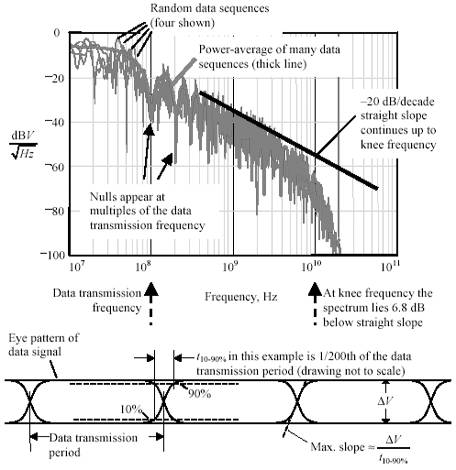At the knee frequency the average spectral power density of a random digital signal with Gaussian rising and falling edges lies 6.8 dB below the straight slope shown in Figure 1.1. Obviously, there is no crisply defined knee at this point. Some authors define the bandwidth of a digital signal as 0.35/ t 10 “90% , which happens to lie at the “3 dB point in Figure 1.1, closer to the meat of the spectrum associated with a rising edge. For some calculations this may produce a more accurate result, however, I still prefer to use [1.3], thinking of it as a conservative over -estimate of bandwidth. If the parasitic elements in my circuit remain insignificant up to [1.3] then I know they can safely be ignored. If, on the other hand, the parasitic elements begin to give difficulty at frequency [1.3] I immediately turn to more accurate techniques (such as Spice, Fourier-Transforms, or Laplace Transforms) to ferret out the exact circuit response.

POINTS TO REMEMBER

• The knee frequency ,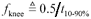Hz, is a crude estimate of the highest frequency content within a particular digital signal.
• The frequency 0.35/ t 10 “90% Hz may better approximate the meat of the spectrum associated with a rising edge.High-Speed Signal Propagation[c] Advanced Black Magic
ISBN: 013084408X
EAN: N/A
Year: 2005
Pages: 163